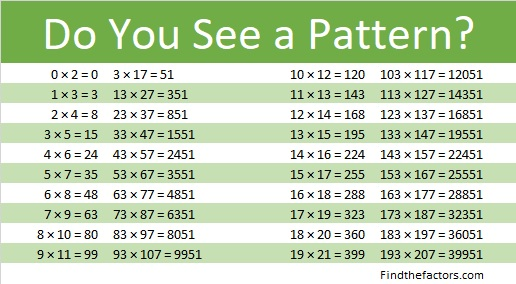# 851 Give This Apple to Your Teacher This Year

This puzzle looks a little like an apple. It’s a level 5 puzzle so it won’t be that easy. If you can solve the puzzle, give it to your teacher!Print the puzzles or type the solution on this excel file: 12 factors 843-852

851 is the hypotenuse of a Pythagorean triple:

• 276-805-851 which is 23 times (12-35-37)

851 is a palindrome in three other bases:

• 353 BASE 16, because 3(16²) + 5(16¹) + 3(16º) = 851
• 191 BASE 25, because 1(25²) + 9(25¹) + 1(25º) = 851
• NN BASE 36 (N is 23 base 10) because 23(36¹) + 23(36º) = 23(37) = 851

Here is 851 factoring information:

• 851 is a composite number.
• Prime factorization: 851 = 23 × 37
• The exponents in the prime factorization are 1 and 1. Adding one to each and multiplying we get (1 + 1)(1 + 1) = 2 × 2 = 4. Therefore 851 has exactly 4 factors.
• Factors of 851: 1, 23, 37, 851
• Factor pairs: 851 = 1 × 851 or 23 × 37
• 851 has no square factors that allow its square root to be simplified. √851 ≈ 171904851 is in this cool pattern:This site uses Akismet to reduce spam. Learn how your comment data is processed.